Essential knowledge in organic chemistry is resonance. Molecules can write a resonance structure if there is a double or triple bond. Electrons don’t stay in one place; they move around in many places. As a result, a single molecule can have various structural formulas.

When learning about resonance, some people may have the image of electrons moving incessantly. However, resonance is not such a concept.

One of the first concepts in organic chemistry that all people learn about is resonance. However, many people don’t understand the concept of resonance and how to write it, and as a result, they dislike organic chemistry in college.

Once you learn the resonance structure, you will be able to understand the concepts of molecular stabilization and acidity. In this section, we will explain the principles of resonance.

## Ozone and Benzene Cannot Be Explained By the Lewis Structure

The Kekule and Lewis structures are frequently used to describe the structure of molecules. The Kekule structure is used to represent molecular bonds as lines. On the other hand, the Lewis structure is used to represent molecular bonds as dots.

The following are the Kekule and Lewis structures.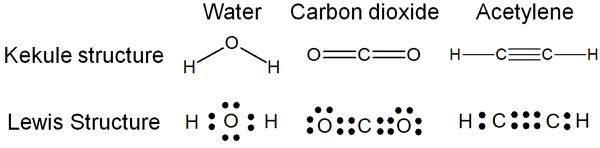In actual organic chemistry, the Kekule structure and the Lewis structure are used in combination.

However, there are some situations that do not make sense when written in a Lewis structure. Commonly used examples include ozone (O3), benzene, and nitrate ion (NO3). The structural formula for each is as follows.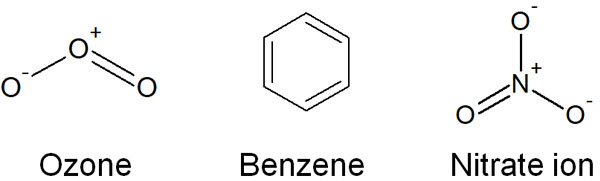For example, let’s look at the ozone. In ozone, there is a single bond and a double bond in the molecule. If this structure is correct, the bonding strength should be stronger at the double bond. In reality, however, the strength of the bonds in ozone is the same for the single and double bonds.

The same is true for benzene and nitrate ions (NO3). Why is this the case? This is because the following structures are mixed in ozone, benzene and nitrate ions.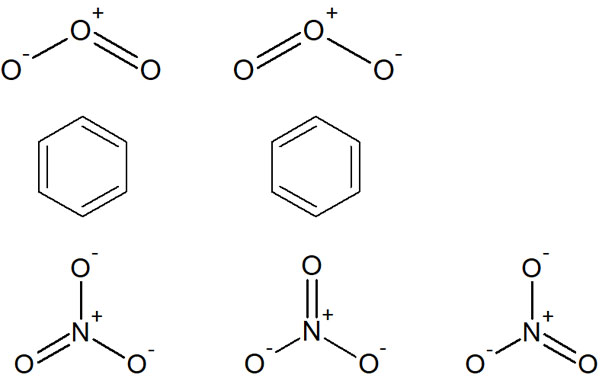For example, in ozone, there is a mixture of two structures. In nitrate ions, there are three structures. Despite the fact that there are multiple structural formulas, writing in a single structural formula leads to inconsistencies.

### Due to Delocalization, Electrons Are Spread Throughout the Molecule

To resolve this inconsistency, we use resonance structures. By writing the movement of electrons, we can write multiple structural formulas. For example, in ozone, we have the following.You can write resonance structures by describing the movement of an unshared electron pair (lone pair). This allows us to understand that there are several structural formulas that are mixed together.

-Resonance Is Not the Movement of Electrons One After Another

It is important to note that resonance does not mean that electrons are moving one after another in the molecule. In writing the resonance structures, the arrows are utilized to describe the movement of electrons. Therefore, some people think that the electrons are constantly moving through the molecule, and the structural formula is changing.

However, this is not true. It is important to understand that resonance is a mixture of multiple structural formulas. Of course, the movement of electrons can change the structural formulae. However, a mixture of several structures is resonance.

Therefore, in ozone, the following is the correct structural formula.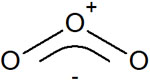In ozone, the two structural formulas exist in a 1:1 ratio. Similarly, in benzene and nitrate ions, multiple structural formulas are mixed in a certain ratio.

-Resonance Causes Electrons to Delocalize

If we could write resonance structures, what would happen to the existence of electrons? Electrons do not stay in one place, but exist in many places. The more resonance structures we can write, the higher the probability that electrons exist in all kinds of places.

This is called the delocalization of electrons. Electrons don’t have to stay in one place; they can be found everywhere in the molecule.

### The More Resonance Structures You Can Write, the More Stable the Electrons Become

What does it mean to write resonance structures? If you can write resonance structures, the electrons become more stable.

It is known that the more electrons go inside a small box, the higher the energy. Electrons are waves, and in quantum mechanics, they are represented by a wave function. In short, you can understand that electrons are waves.

Even though electrons are waves, there are waves of various widths (frequencies). Suppose we confine these waves to a small area. Then waves of various frequencies (waves with various motions) have to be confined to a small area, resulting in high energy.

On the other hand, if we can write several structural formulas using resonance structures, then the range in which the electrons can exist becomes larger. Delocalization allows electrons to move freely within the molecule instead of being confined to a small area.

As a result of expanding the area where the electrons can exist, the electrons become more stable. This is the reason why the more resonance structures can be written, the more stable the electrons become.

• Being able to write resonance structures = Stabilization of electron

We are taught that if we can write resonance structures, the electrons become more stable, but we rarely have a chance to think about the reason. Normally, we would have to explain the reason for electron stabilization using difficult mathematical formulas, but this would make the explanation very difficult. Therefore, only physics students who are interested in quantum mechanics should try to figure out the reasons while solving the equations.

## The Presence of a Double Bond (pi Bond) Causes Resonance

How does resonance occur? Roughly, consider that you can write resonance structures when there is a double bond.

In molecular bonding, the s and p orbitals are involved in the bond. In the case of a single bond (σ-bond), it is not possible to write a resonance structure. On the other hand, if there is a pi bond as a double or triple bond, resonance structures can be written.

Ozone, benzene, and nitric acid all have one thing in common. They all have a double bond in their molecules. Let’s think of it as having a double bond or a triple bond that allows us to draw resonance structures.

### The Octet Rule Contributes to a Stable Structure

Does any molecule resonate if it has a π bond? Of course, this is not the case. Even if you can write resonance structures, it is meaningless if the structures are not stable. To be more precise, we need to write resonance structures that satisfy the octet rule.

The stability of an atom with eight electrons is called the octet rule. In particular, carbon, nitrogen, oxygen, and fluorine atoms must satisfy the octet rule.

For example, if the electrons are free to move, we can write the following resonance structure.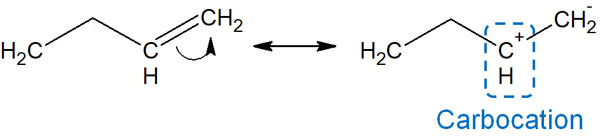But in practice, this resonance does not occur. This is because it produces a carbocation. In a carbocation, there are only six electrons in a carbon atom. If there are eight valence electrons, the octet rule is satisfied, and the carbocation is stable. The carbocation, however, does not satisfy the octet rule, and is unstable.

In other words, this type of resonance almost never occurs. Even if there is a double bond (pi bond), it does not always resonate.

On the other hand, if resonance structures can be written to satisfy the octet rule, the electrons are delocalized. Such resonances can be said to make a significant contribution to structural stabilization.

## The Presence of Oxygen and Nitrogen Atoms and Conjugated Structures Is Important for the Resonance Structure

What makes a molecule resonate? Is there a way to find it? We mentioned above that the presence of π-bonds (double or triple bonds) is a prerequisite for resonance. In addition, it is easy to find resonance when the following conditions are met.

• The presence of an oxygen or nitrogen atom.
• There’s a conjugate structure.

In fact, there are a number of other conditions that can cause resonance. For example, the presence of atoms such as phosphorus (P) and sulfur (S) will cause resonance. However, for the sake of clarity, let’s focus on these conditions first.

If you can write resonance structures, in many cases, there is an oxygen atom (O) or a nitrogen atom (N) in the molecule. These atoms have unshared electron pairs and can make double bonds. They can also be positively or negatively charged. Since they can change their properties, they can easily become the starting point for resonance.

However, even if only oxygen or nitrogen atoms are present, it is not possible to write resonance structures; double bonds are required. In particular, when there is a conjugated structure, it is possible to write resonances. Conjugation is a structure that consists of a series of double bonds. For example, the benzene ring is a kind of conjugate.

The following is the resonance structure of aniline.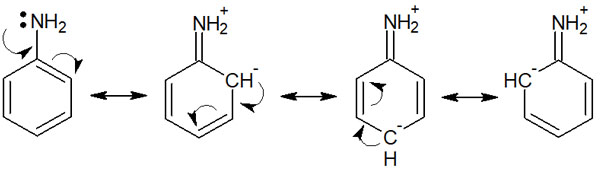In this way, we can draw resonance structures in aniline. Starting from the electrons of the nitrogen atom, multiple resonances can be drawn using the conjugated structure of the aromatic ring (benzene ring).

Of course, even without the conjugated structure, there are cases where multiple resonances can be drawn, such as nitrate ions (NO3) and carbonate ions (CO32-). But in any case, you can write resonances, starting from an oxygen or nitrogen atom, and using double or triple bonds.

### Resonances Stabilize Molecules and Are Related to Acidity and Basicity

What can we learn by writing these resonances? As we explained earlier, the more resonance structures we can write, the more delocalization of the molecule occurs, and the more stable the molecule becomes. Although the octet rule needs to be satisfied, the wide distribution of electrons allows the molecule to exist in a stable state.

This plays a role in the acidity and basicity of the molecule.

For example, a hydroxy group (-OH) is a neutral molecule. It is neither acidic nor basic. Phenol, on the other hand, is known to be an acidic molecule.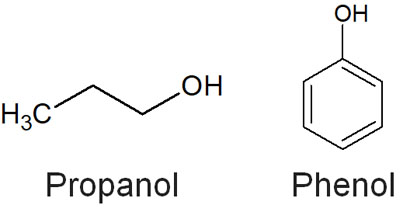For phenol, which has -OH bonded to the benzene ring, why is it not neutral like the hydroxy group bonded to the alkyl chain? In phenol, the resonance structures can be written by ionization. It is as follows.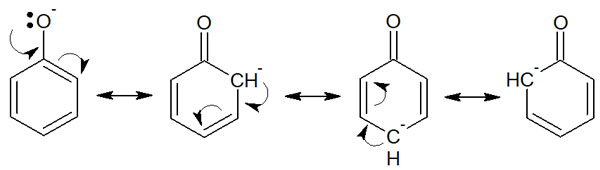In the case of propanol, it is not possible to write resonance structures because it is all single bonds (σ-bond). On the other hand, in the case of phenol, after ionization, it is possible to write multiple resonance structures using the benzene ring. In other words, the structure stabilizes after ionization. This is the reason why phenol shows acidity.

The fact that a compound is stabilized by resonance is related to its acidity. In high school chemistry, we just learn that phenol is acidic, but we don’t understand why. So if you learn about resonance, you will be able to understand why phenol is acidic while the hydroxy group (-OH) attached to the alkyl chain is neutral.

## Resonant Structures Are the Basis of Organic Chemistry

A concept that is basic but difficult to understand is resonance. When first learning about resonance, many people wonder why electrons move on their own. As a result, we lose our understanding of organic chemistry.

In some cases, such as ozone, there are several structures that exist in more than one. So by writing resonance structures, we need to understand that there are multiple molecules mixed together. By writing resonances, we can show that the electrons are not constantly moving one after another, but that they exist in a wide range.

In general, however, you need to write the resonances in such a way that they satisfy the octet rule. If you understand this rule and focus on the pi bonds (double and triple bonds) and the oxygen and nitrogen atoms, you will be able to distinguish whether or not you can draw resonance structures.

Learning resonance structures is useful when understanding synthetic reactions. Without understanding the concept of resonance, it is impossible to understand the reaction mechanisms of organic chemistry. Therefore, it is important to understand the concept of resonance and to be able to write resonance structures.